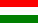This application brings for you azeotropic data (azeotrope boiling point/temperature and composition), based on vapor-liquid equilibrium calculations. So the program does not provide stored experimental data, but predicts them theoretically.

## Method of calculation

At an azeotropic point the composition of vapor- and liquid phases are identical (xi = yi), and the bubble- and dew point curves have a local extrema.

Take an objective function that measures the relative deviation of the compositions between the vapor- and liquid phases:

fi = (xi - yi) / xi

At every x points in the composition space, where fi = 0 must be an azeotropic point. (Except the corner points representing the pure components (i), where xi = 1 and xj≠i = 0.) The program uses multi-variate Newton-Raphson search to locate these points.

The composition of the vapor phase is calculated according to the modified Raoult's law:

pi = γi  psat.,i  xi

yi = pi / Σj=1→N pj

where:
• pi is the partial pressure of component i in the vapor phase of the mixture
• γi (gamma) is the activity coefficient of component i in the liquid mixture
• psat.,i is the vapor pressure of saturated pure component i
• x is the liquid phase mole fraction of component i
• y is the vapor phase mole fraction of component i
The program uses the UNIFAC (modified, Dortmund) model for calculating the activity coefficient.